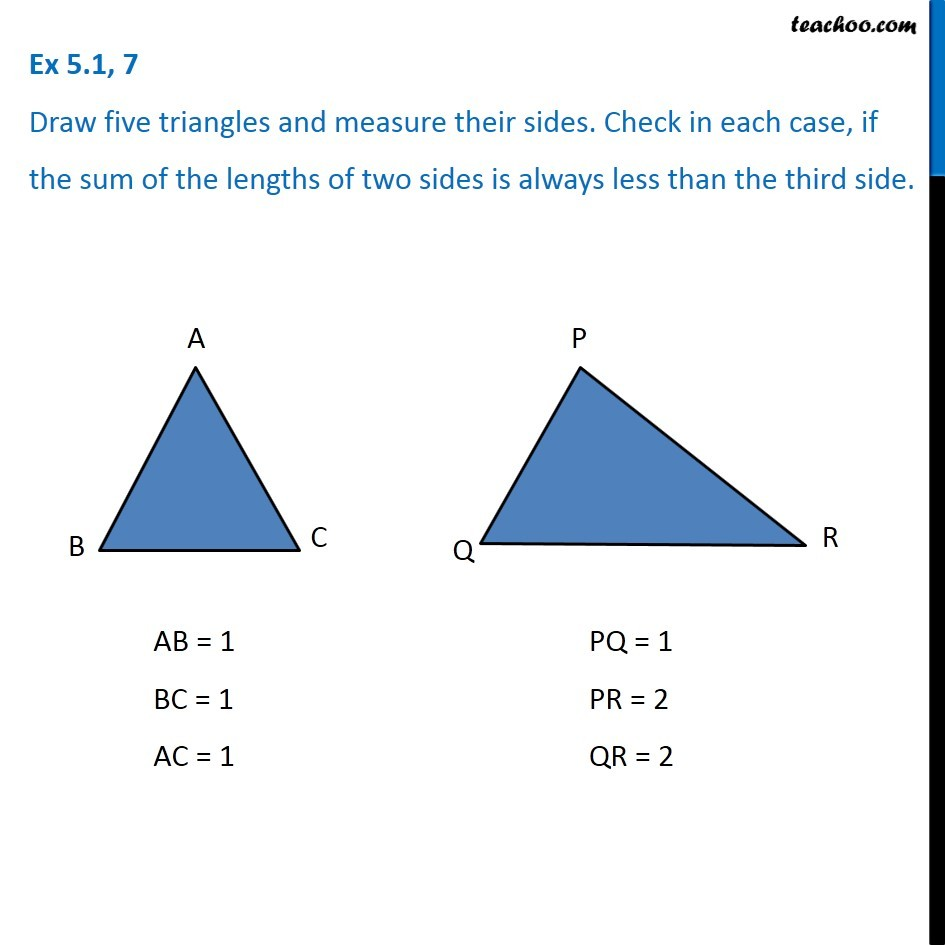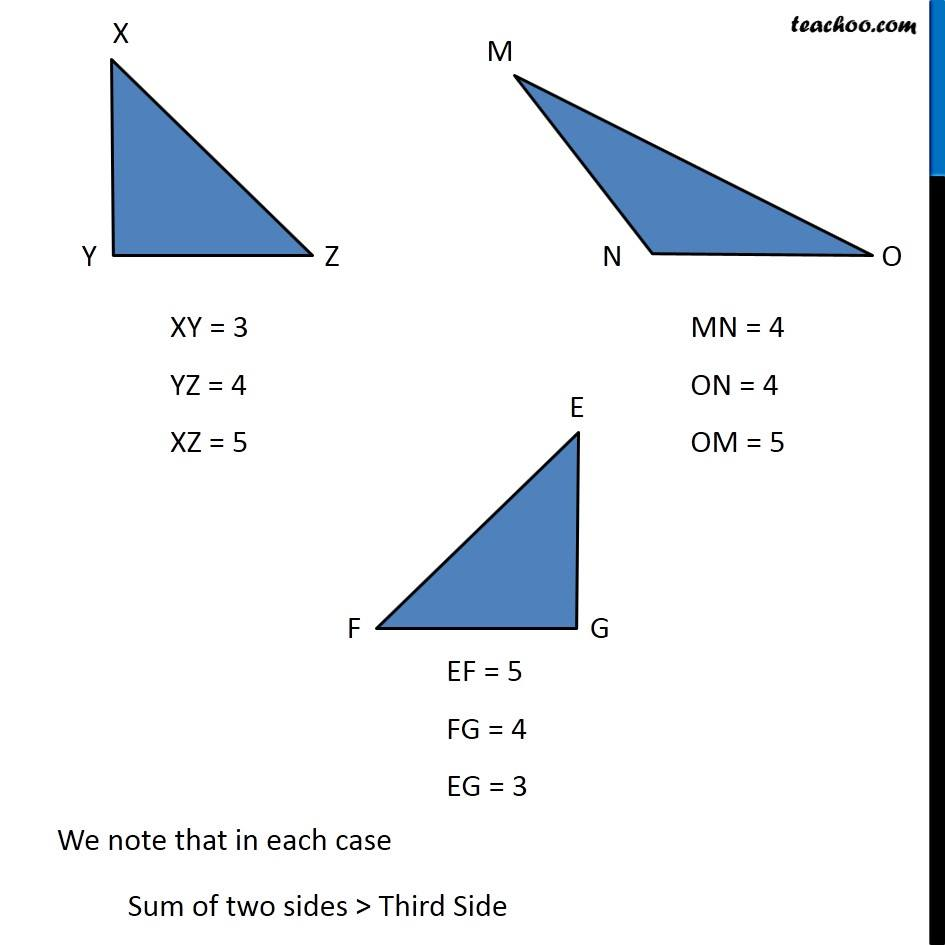Measuring Line Segments

Chapter 5 Class 6 Understanding Elementary Shapes
Concept wise### Transcript

Ex 5.1, 7 Draw five triangles and measure their sides. Check in each case, if the sum of the lengths of two sides is always less than the third side. We take 5 triangles with sides > All equal > 2 equal, 1 different > All 3 unequal (right angle, acute, obtuse angled triangle) And verify that in each case Sum of two sides > Third Side